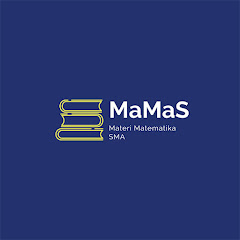# MaMaS : Materi Matematika SMA

1K+Everyone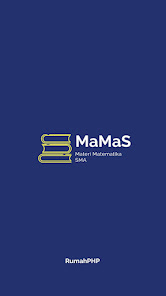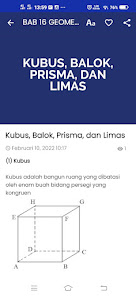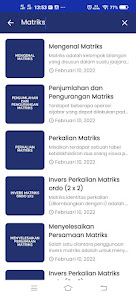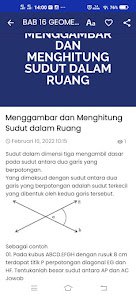MaMas contains Complete High School / Vocational / MA Mathematics Materials, from Compulsory Mathematics and Specialized Mathematics. Systematics of material based on the Latest Revised National Curriculum (Revised 2018). For those who use the old curriculum, the material remains the same but the systematics are not sequential so you can choose the material according to your needs.
The material consists of the following material.

MATH MANDATORY
(Mathematics SMA/MA/SMK All Majors)

Class X
1. Absolute Value Equations and Inequality
2. System of Linear Equations
3. Relations and Functions
4. Composition and Inverse of Functions
5. Comparison and Trigonometric Functions
6. Sine and Cosine Rules

Class XI
1. Mathematical Logic
2. Mathematical Induction
3. Linear Program
4. Limits of Algebraic Functions
5. Matrix
6. Rows and Rows
7. Derivatives of Algebraic Functions
8. Indefinite Integral Algebraic Functions

Class XII
1. Transformation
2. Geometry of Space
3. Field Geometry
4. Counting Rules and Probability
5. Statistics

MATHEMATICS
(Mathematics SMA/MA Majoring MIPA/IPA)

Class X
1. Systems of Linear and Quadratic Equations
2. Linear and Quadratic Inequality Systems
3. Exponents and Logarithms
4. Exponential and Logarithmic Equations and Functions
5. Absolute, Fractional and Irrational Inequality
6. Vector

Class XI
1. Trigonometric Formulas
2. Trigonometric Equations and Functions
3. Polynomials (Many Terms)
4. Cone Slice
5. Circle
6. Of course integrals of algebraic functions

Class XII
1. Infinite Limits of Algebraic Functions and Limits of Trigonometry
2. Derivatives of Trigonometric Functions
3. Application of Function Derivatives
4. Indeterminate Integrals of Trigonometric Functions
5. Integral with Partial Integral Substitution Rule
6. Integral of Exponential and Logarithmic Functions
7. Inferential Statistics
8. Financial Mathematics
Updated on
Feb 16, 2022

## Data safety